RRB Group D Mock Test - 4

# RRB Group D Mock Test - 4

Test Description

## 100 Questions MCQ Test RRB (Group D) - Mock Tests & Previous Year Papers | RRB Group D Mock Test - 4

RRB Group D Mock Test - 4 for Railways 2023 is part of RRB (Group D) - Mock Tests & Previous Year Papers preparation. The RRB Group D Mock Test - 4 questions and answers have been prepared according to the Railways exam syllabus.The RRB Group D Mock Test - 4 MCQs are made for Railways 2023 Exam. Find important definitions, questions, notes, meanings, examples, exercises, MCQs and online tests for RRB Group D Mock Test - 4 below.
Solutions of RRB Group D Mock Test - 4 questions in English are available as part of our RRB (Group D) - Mock Tests & Previous Year Papers for Railways & RRB Group D Mock Test - 4 solutions in Hindi for RRB (Group D) - Mock Tests & Previous Year Papers course. Download more important topics, notes, lectures and mock test series for Railways Exam by signing up for free. Attempt RRB Group D Mock Test - 4 | 100 questions in 90 minutes | Mock test for Railways preparation | Free important questions MCQ to study RRB (Group D) - Mock Tests & Previous Year Papers for Railways Exam | Download free PDF with solutions
 1 Crore+ students have signed up on EduRev. Have you?
RRB Group D Mock Test - 4 - Question 1

### A is two years older than b who is twice as old as C. If the total of the ages of A, B and C be 27, then how old is B?

Detailed Solution for RRB Group D Mock Test - 4 - Question 1 Let C's age be x years
Then, B's age = 2x years
A's age = (2x + 2) years
∴ (2x + 2) + 2x + x = 27
⇒ 5x = 25
⇒ x = 5
Hence B's age = 2x = 10 years
RRB Group D Mock Test - 4 - Question 2

### Which one of the following pairs of equations has a unique solution?

Detailed Solution for RRB Group D Mock Test - 4 - Question 2

Given two equations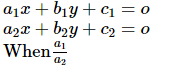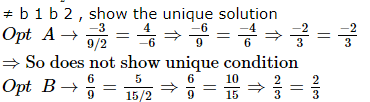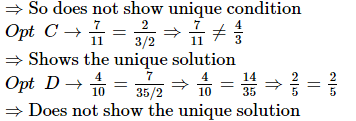RRB Group D Mock Test - 4 - Question 3

### One side of a right triangle measures 12 cm and the difference between the hypotenuse and the other side is 8 cm. The area of the triangle is

Detailed Solution for RRB Group D Mock Test - 4 - Question 3

Let the hypotenuse = x cm
Then the other side is (x - 8) cm
By Pythagoras theorem
(x - 8)2 + (12)2 = x2
⇒ x2 + 64 - 16x + 144 = x2
⇒ -16x = -208
⇒ x = 208/16 = 13 cm
∴ Hypotenuse = 13cm
so the other side = (13 - 8) = 5cm
Area = 1/2 x 12 x 5
= 30cm2

RRB Group D Mock Test - 4 - Question 4
The average weight of three men A,B and C is 84 kg. D joins them and the average weight of the four becomes 80 kg. If E, whose weight is 3 kg more than that of D,replaces A,the average weight of B,C,D and E becomes 79 kg. The weight of A is
RRB Group D Mock Test - 4 - Question 5
Who wrote 'Vaishnav Jan te.....'?
RRB Group D Mock Test - 4 - Question 6

A motor boat whose speed is 15 km/hr in still water goes 30 km downstream and comes back in 4 hrs and and 30 minutes. Determine the speed of the stream.

Detailed Solution for RRB Group D Mock Test - 4 - Question 6

Let x km/hr be the speed of water
The speed of motor boat in still water is 15 km/hr, therefore, its speed downstream is (15 + x) km/hr and the speed upstream is (15 - x) km/hr
Time taken for given 30 km downstream = 30/15 + x hours
Time taken for going 30 km upstream = 30/15 - x hours
Since the total time is given to be 4 hours 30 minutes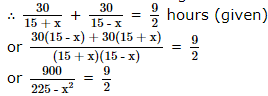or 1800 = 9(225 - x2)
or 200 - 225 + x2 = 0
or x2 = 25
⇒ x = 5, - 5
∴ x = 5 [as x cannot be negative so x = -5 is rejected]
Hence the speed of water = 5 km/hr

RRB Group D Mock Test - 4 - Question 7
A is the father of C. But C is not his son.
E is the daughter of C. F is the spouse of A.
B is the brother of C. D is the son of B.
G is the spouse of B. H is the father of G.
Who is the Grand mother of D?
RRB Group D Mock Test - 4 - Question 8
Which one of the following statements is correct on the basis of Census-2001 ?
RRB Group D Mock Test - 4 - Question 9
Choose the analogous pair.
Pedant : Erudition
RRB Group D Mock Test - 4 - Question 10
Choose the numeral pair which is different from others .
Detailed Solution for RRB Group D Mock Test - 4 - Question 10 This is the only pair in which the second number is twice the first one
RRB Group D Mock Test - 4 - Question 11
If 'nitco sco tingo' stands for 'softer than flower'; 'tingo rho mst' stands for 'sweet flower fragrance' and 'mst sco tmp' stands for 'sweet than smile', what would 'fragrance' stand for ?
RRB Group D Mock Test - 4 - Question 12
Complete the analogous pair.
Calf : Cow : : Puppy : ?
RRB Group D Mock Test - 4 - Question 13

The compound interest on Rs 10000 in 2 years at 4% per annum, the interest being compounded half-yearly, is

Detailed Solution for RRB Group D Mock Test - 4 - Question 13

P = 10,000
T = 2 years
R = 4% (compounded half yearly)
⇒ R% = 2 + 2 + 4/100 = 4 + 0.04
⇒ R% = 4.04%
⇒ CI = P[1 + R/100 ]T - P
⇒ CI = 10000 [1 + 4.04/100 ]2 - 10000
⇒ CI = (104.04)2 - 10000
⇒ CI = 10824.3216 - 10000
⇒ CI = Rs 824.3216

RRB Group D Mock Test - 4 - Question 14
The main cause of the punic wars was :
RRB Group D Mock Test - 4 - Question 15
How many rectangles are there in the figure below?
Detailed Solution for RRB Group D Mock Test - 4 - Question 15 The figure in question has been labelled as under:

It is clear from the diagram above that there are eight rectangles namely
AEFM, EGHF, AGHM, GBLK, KLCN, GBCN, MHND, ABCD
RRB Group D Mock Test - 4 - Question 16
What is China's currency called?
RRB Group D Mock Test - 4 - Question 17
Who is the author of the book 'A Foreign Policy of India'?
RRB Group D Mock Test - 4 - Question 18
The Nehru-Liaquat Pact between India and Pakistan was signed in 1950 to resolve the issue of:
RRB Group D Mock Test - 4 - Question 19
Four positions of a dice are shown below. What number must be at the bottom face when the dice is in the position as shown in fig. (III)?
Detailed Solution for RRB Group D Mock Test - 4 - Question 19 From figure (I), (II), (IV) we conclude that 5, 6, 1 and 2 lie adjacent to 4.
Hence, 3 must lie opposite 4 and vice-versa. In fig. (III), 3 is at the top and consequently 4 must lie at the bottom face.
RRB Group D Mock Test - 4 - Question 20
Soni travelled 9 km to the west, then turned right and travelled 7 km, then turned left and travelled 8 km, then turned back and travelled 11km, then turned right and travelled 7 km. How far is he from the starting point?
RRB Group D Mock Test - 4 - Question 21
According to the latest statistics of the government, what percent of growth rate has been registered by the banking sector in December 2017 post Centre’s PSB recapitalisation plan?
RRB Group D Mock Test - 4 - Question 22
Who was appointed the Managing Director (MD) and CEO of National Payments Corporation of India (NPCI)?
RRB Group D Mock Test - 4 - Question 23
Who is known as Indian Bismarck?
RRB Group D Mock Test - 4 - Question 24
'Kanchipuram' is in which of the following states?
RRB Group D Mock Test - 4 - Question 25
What are the two main parts of the computers required to be present essentially?
RRB Group D Mock Test - 4 - Question 26
Which of the following is a national net work
RRB Group D Mock Test - 4 - Question 27
GDP at factor cost is-
RRB Group D Mock Test - 4 - Question 28
National Income is the :
RRB Group D Mock Test - 4 - Question 29
The minor form of condensation near the surface that forms a mass is :
RRB Group D Mock Test - 4 - Question 30
Indicate the wrong combination :
RRB Group D Mock Test - 4 - Question 31
The Indian civil Service was started during the tenure of
RRB Group D Mock Test - 4 - Question 32
In ancient India historical geography, the name 'Ratnakara' denoted
RRB Group D Mock Test - 4 - Question 33
Which one of the following species is obtained from flower buds?
RRB Group D Mock Test - 4 - Question 34
Which one of the following is Bragg's equation used for the study of crystals?
RRB Group D Mock Test - 4 - Question 35
Which of the following is used for writing on glass?
RRB Group D Mock Test - 4 - Question 36
Which one of the follwing is not an official language of the United Nations?
RRB Group D Mock Test - 4 - Question 37
Which one of the following organisms can serve as a biofertilizer for rice crop?
RRB Group D Mock Test - 4 - Question 38
Which one of the following is in the correct sequential order in which these appeared under simulated primitive earth conditon?
RRB Group D Mock Test - 4 - Question 39
Consider the figure of a fountain with four holes given below:

Which one of the holes in the fountain will throw the water farthest?
RRB Group D Mock Test - 4 - Question 40
The changing composition of the export trade is indicative of structural transformation of Indian economy in favour of modernisation. The best indicator of this trend is the :
RRB Group D Mock Test - 4 - Question 41
Barium in a suitable form is administered to patients before an X-ray examination of the stomach, because
RRB Group D Mock Test - 4 - Question 42

The acceleration due to gravity of a catastropic earthquake will be

RRB Group D Mock Test - 4 - Question 43
With reference to the latest developments in stem cell research, consider the following statements :
1. The only source of human stem cells are the embryos at blastocyst stage.
2. The stem cells can be derived without causing destruction to blastocysts.
3. The stem cells can regenerate themselves in vitro virtually forever.
4. Indian research centres also created a few cell lines which can be developed into many types of tissues.
Which of these statements are correct?
RRB Group D Mock Test - 4 - Question 44
Which of the following would lead to amlnutrition?
1. Overnutrition
2. Undernutrition
3. Imbalanced nutrition
Select the correct answer by using the codes given below:
RRB Group D Mock Test - 4 - Question 45
A rectangular box is open on one side. The number of pairs of parallel planes of such a box is :
RRB Group D Mock Test - 4 - Question 46
The UNESCO has recently included the “bird language”, prevalent in which country, in its list of Intangible Cultural Heritage citing it as an endangered part of world heritage?
RRB Group D Mock Test - 4 - Question 47
Find the missing character.
Detailed Solution for RRB Group D Mock Test - 4 - Question 47 Clearly, the number inside the circle is equal to the sum of the product of the upper three numbers and the product of the lower three numbers. Thus,ans is 130
RRB Group D Mock Test - 4 - Question 48

Nawab Sanaullah Zehri, recently resigned before a no-confidence motion was taken against him in the provincial assembly. He belonged to which province of Pakistan?

RRB Group D Mock Test - 4 - Question 49
Laparoscopy is connected with
RRB Group D Mock Test - 4 - Question 50

How many such pairs of letters are there in the word MODERATE each of which has as many letters between them in the word as in the English alphabet?

Detailed Solution for RRB Group D Mock Test - 4 - Question 50

There are 4 pairs in the word 'MODERATE' i.e.
(1) O and R - 2 letters between them - D,E
In the alphabat series also there are 2 letters between O and R i.e. P,Q
(2) D and A - 2 letters between them - E, R
In the alphabat series there are 2 letters between D and A i.e. C,B
(3) O and T - 4 letters between them - DERA
In the alphabat series there are 4 letters between O and T i.e. P,Q, R, S
(4) R and T - 1 letters between them - A
In the alphabat series there are 1 letter between R and T i.e. S
So the CORRECT ANSWER is More than 3 i.e. (E)

RRB Group D Mock Test - 4 - Question 51

What should come in the place of question - mark (?) in the following series?
YA, CW, ?, GS, QI

Detailed Solution for RRB Group D Mock Test - 4 - Question 51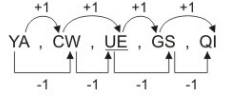RRB Group D Mock Test - 4 - Question 52
Arrange the words given below in a meaningful sequence.
1. Post-box 2. Letter 3. Envelope 4. Delivery 5. Clearance
RRB Group D Mock Test - 4 - Question 53

If + means x, ÷ means -, x means ÷ and - means +
what will be the value of 4 + 11 ÷ 5 - 55 = ?

Detailed Solution for RRB Group D Mock Test - 4 - Question 53

Given expression : = 4 x 11 - 5 + 55 = 44 - 5 + 55 = 94

RRB Group D Mock Test - 4 - Question 54
In a mixture of 60 litres, the ratio of milk and water is 2:1. If the ratio of milk and water is to be 1:2, then the amount of water to be further added must be
RRB Group D Mock Test - 4 - Question 55
Union Home Ministry has declared which state as “disturbed area” for six more months (till June 2018) under Armed Forces (Special Powers) Act – AFSPA?
RRB Group D Mock Test - 4 - Question 56
Which among the following states has become the second north eastern state after Sikkim to be declared Open Defecation Free?
RRB Group D Mock Test - 4 - Question 57
Complete the analogous pair.
64 : 8 :: 16 : 4 :: ? : 2
RRB Group D Mock Test - 4 - Question 58
What will come in place of question mark (?) in the following series.
33, 28, 24, ?, 19, 18
RRB Group D Mock Test - 4 - Question 59

Two numbers x and y(x>y) are such that their sum is equal to three times their difference. Then the value of 3xy/2(x- y2) will be

RRB Group D Mock Test - 4 - Question 60
Atul and Babita enter into a business partnership in which Atul contributes Rs 2000 for 9 months and Babita contributes Rs 5000 for 7 months. A profit of Rs 1100 will be divided between Atul and Babita in the ratio of
RRB Group D Mock Test - 4 - Question 61
60% of the students in a school are boys.If the number of girls in the school is 300,then the number of boys is
RRB Group D Mock Test - 4 - Question 62
Who has been sworn in as the Deputy National Security Adviser (NSA) by the Union Government?
RRB Group D Mock Test - 4 - Question 63
The ‘Karnataka International Travel Expo’ (KITE), considered the “largest” B2B travel event in India, will be held in which city of Karnataka?
RRB Group D Mock Test - 4 - Question 64
If 15 pumps of equal capacity can fill a tank in 7 days. Then how many extra pumps will be required to fill the tank in 5 days?
RRB Group D Mock Test - 4 - Question 65
A train 132 m long passes a telegraph pole in 6 secs. Find the speed of the train.
RRB Group D Mock Test - 4 - Question 66
Profit after selling a commodity for Rs 425 is same as loss after selling it for Rs 355,the cost of the commodity is:
RRB Group D Mock Test - 4 - Question 67
In a row of trees, one tree is fifth from either end of the row. How many trees are there in the row ?
Detailed Solution for RRB Group D Mock Test - 4 - Question 67 number of trees in the row = ( 4 + 1 + 4 ) = 9.
RRB Group D Mock Test - 4 - Question 68

A bag contain Rs 102 in the form of rupee, 50-paise and 10-paise coins in the ratio 3:4:10. The number of 10-paise coins is

Detailed Solution for RRB Group D Mock Test - 4 - Question 68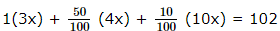⇒ 300x + 200x + 100x = 102 x 100
⇒ 600x = 1020 x 10
⇒ x = 17
Hence, number of 10 paisa coins are = 10(17) = 170 coins

RRB Group D Mock Test - 4 - Question 69
At which of the following places did Gautam Budha attain Nirvana?
RRB Group D Mock Test - 4 - Question 70
Which space agency, has announced that it would launch two missions – GOLD and ICON – during 2018 to explore the little-understood area of 96 km above Earth’s surface?
RRB Group D Mock Test - 4 - Question 71
When a vehicle passes, TV reception gets distorted. This is because
RRB Group D Mock Test - 4 - Question 72
A fuse wire is used for :
RRB Group D Mock Test - 4 - Question 73
Which of the following statements regarding cellulose are correct ? (1) It is a naturally occurring organic substance found in plants (2) It is used for making rayon (3) It consists of long unbranched chain of glucose units
RRB Group D Mock Test - 4 - Question 74
RRB Group D Mock Test - 4 - Question 75
Which of the following machines will fail to give satisfactory result in vacuum ?
RRB Group D Mock Test - 4 - Question 76
Food remains unspoilt in a refrigerator for a longer time because
RRB Group D Mock Test - 4 - Question 77
Sleeping under a tree at night is dangerous since :
RRB Group D Mock Test - 4 - Question 78

A sum of money lent out at simple interests amounts to Rs. 720 after 2 years and to Rs. 1020 after a further period of 5 years. The sum is :

Detailed Solution for RRB Group D Mock Test - 4 - Question 78

Principal + SI for 2 years = 720 Rs. ……(I)
Principal + SI for 7 years = 1020 Rs. ……(II)
Subtracting equation (I) from (II),
S.I. for 5 years = (1020 – 720) = 300 Rs.
∴ S.I. for 2 years = 300 x 2/5 = 120 Rs
∴ Principal = (720 – 120) = 600 Rs.

RRB Group D Mock Test - 4 - Question 79
83% of 621 = ?
RRB Group D Mock Test - 4 - Question 80

If pxq = p+q+(p/q), then the value of 8x2 is

RRB Group D Mock Test - 4 - Question 81
For which sport is the Val Barker Cup awarded in the Olympic Games?
RRB Group D Mock Test - 4 - Question 82
Name the player to win the Men’s 10m Air Pistol Gold at the 61st National Shooting Championship (NSCC)?
RRB Group D Mock Test - 4 - Question 83
Which country hosted the Hopman Cup 2018?
RRB Group D Mock Test - 4 - Question 84
Below is given statement followed by two conclusions numbered I and II. You have to consider the statement and the following conclusions and decide which of the conclusions is follows in the statement :
Statement :
Some infrastructure in transportation was made by the British in India but its aim was to facilitate trade to their advantage.
Conclusions :
I. Foreign rule is always exploitative.
II. Transport infra-structure is necessary to facilitate trade.
RRB Group D Mock Test - 4 - Question 85
A car covers 420 km with a constant speed. If its speed were 10 km/hr more, it would have taken one hour less to cover the distance. The speed of the car is
RRB Group D Mock Test - 4 - Question 86

A alone can do a piece of work in 6 days and B alone in 8 days. A and B undertook to do it for Rs. 3200. With the help of C, they completed the work in 3 days. How much is to be paid to C?

Detailed Solution for RRB Group D Mock Test - 4 - Question 86

C's 1 day's work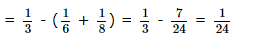A's wages : B's wages : C's wages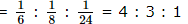∴ C's share (for 3 days) =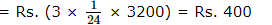RRB Group D Mock Test - 4 - Question 87
The maximum value of sin θ cos θ is
RRB Group D Mock Test - 4 - Question 88

What is the total surface area of a right circular cone of height 14 cm and base radius 7 cm?

RRB Group D Mock Test - 4 - Question 89
Choose one word which cannot be made from the letters of the given word.
EXAMINATION
RRB Group D Mock Test - 4 - Question 90
Which organ of the United Nations has ceased to be operational?
RRB Group D Mock Test - 4 - Question 91

Each of the following questions consists of two sets of figures. Figures 1,2,3 and 4 constitute the Problem Set while figures A,B,C,D and E constitute the Answer Set. There is a definite relationship between figures 1 and 2. Establish a similar relationship between figures 3 and 4 by selecting a suitable figure from the Answer Set .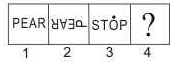RRB Group D Mock Test - 4 - Question 92

In the following problem, out of the five figures marked 1, 2, 3, 4 and 5, four are similar in a certain manner. However, one figure is not like the other four. Choose the figure which is different from the rest.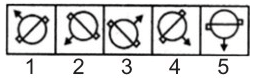RRB Group D Mock Test - 4 - Question 93

In the following questions, select a figure from amongst the four alternatives, which when placed in the blank space of fig. (X) would complete the pattern.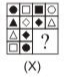RRB Group D Mock Test - 4 - Question 94

P,Q,R,S,T,V,W and Z are sitting around a circle facing the center. R is second to the left of Z who is third to the left of P. T is third to the right of W who is not an immediate neighbour of either R or Z. S is fourth to the right of Z. Q is fourth to the right of T.

Q. In which of the following pairs is the first person sitting to the immediate left of the second person?

Detailed Solution for RRB Group D Mock Test - 4 - Question 94

The seating arrangement is as follows :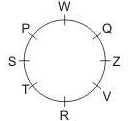RRB Group D Mock Test - 4 - Question 95

Study the following diagram.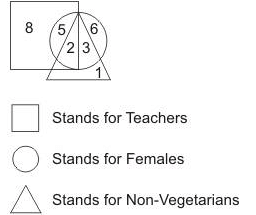Q. Which number in the above figure denotes Vegetarian Females who are not Teachers.

RRB Group D Mock Test - 4 - Question 96

Find out from amongst the four alternatives as to how the pattern would appear when the transparent sheet is folded at the dotted line.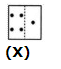RRB Group D Mock Test - 4 - Question 97

Below are the statements followed by two conclusions numbered I and II. You have to consider the statements and the following conclusions and decide which of the conclusion(s) follows the statement(s).

Statements :
a. All books are ledgers.
b. All pens are keys.

Conclusions :
I. Some ledgers are keys.
II. Some keys are books.

Detailed Solution for RRB Group D Mock Test - 4 - Question 97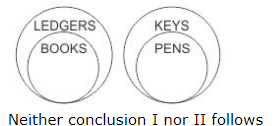RRB Group D Mock Test - 4 - Question 98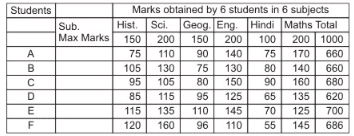Above tables shows the marks obtained by 6 students in 6 different subjects. Observe the table carefully and answer the questions.

Q. What is the percentage difference between the marks gained by the student A in History and C in Hindi?

Detailed Solution for RRB Group D Mock Test - 4 - Question 98

Required % = 90% - 50% = 40%

RRB Group D Mock Test - 4 - Question 99Above tables shows the marks obtained by 6 students in 6 different subjects. Observe the table carefully and answer the questions.

Q. The marks obtained by B in Mathematics is how many times the percentage of marks obtained by F in Science?

Detailed Solution for RRB Group D Mock Test - 4 - Question 99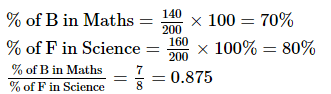Hence % of B in Maths is equal to 0.875 times of % of F in science.

RRB Group D Mock Test - 4 - Question 100Above tables shows the marks obtained by 6 students in 6 different subjects. Observe the table carefully and answer the questions.

Q.  For D what is the difference between the percentage of marks in English and the average percentage of marks in all the subjects?

Detailed Solution for RRB Group D Mock Test - 4 - Question 100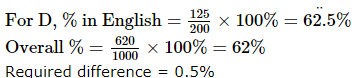## RRB (Group D) - Mock Tests & Previous Year Papers

1 docs|48 tests
 Use Code STAYHOME200 and get INR 200 additional OFF Use Coupon Code
Information about RRB Group D Mock Test - 4 Page
In this test you can find the Exam questions for RRB Group D Mock Test - 4 solved & explained in the simplest way possible. Besides giving Questions and answers for RRB Group D Mock Test - 4, EduRev gives you an ample number of Online tests for practice

1 docs|48 tests